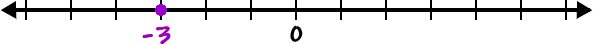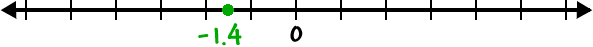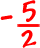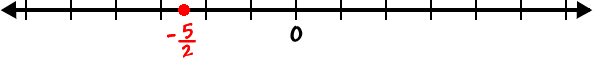Before we get into comparing numbers, let's do some practice with finding negative numbers on a number line.

Let's find -3 on the number line:Let's find -1.4 on the number line:Let's findon the number line: